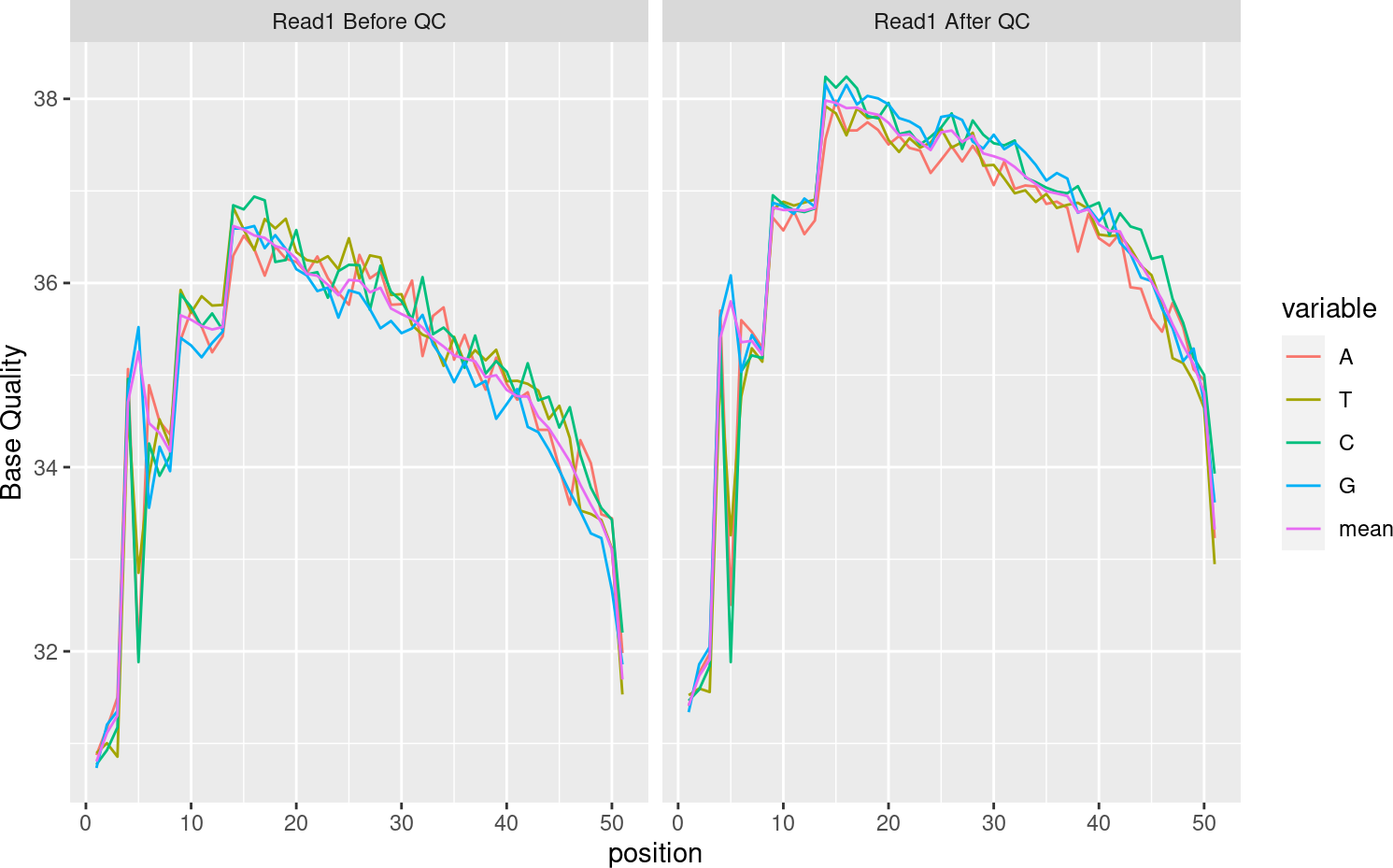# 1 Introduction

The Rfastp package provides an interface to the all-in-one preprocessing for FastQ files toolkit fastp(Chen et al. 2018).

# 2 Installation

Use the `BiocManager` package to download and install the package from Bioconductor as follows:

``````if (!requireNamespace("BiocManager", quietly = TRUE))
install.packages("BiocManager")
BiocManager::install("Rfastp")``````

If required, the latest development version of the package can also be installed from GitHub.

``````BiocManager::install("remotes")
BiocManager::install("RockefellerUniversity/Rfastp")``````

``library(Rfastp)``

# 3 FastQ Quality Control with rfastp

The package contains three example fastq files, corresponding to a single-end fastq file, a pair of paired-end fastq files.

``````se_read1 <- system.file("extdata","Fox3_Std_small.fq.gz",package="Rfastp")
outputPrefix <- tempfile(tmpdir = tempdir())``````

## 3.1 a normal QC run for single-end fastq file.

Rfastp support multiple threads, set threads number by parameter `thread`.

``````se_json_report <- rfastp(read1 = se_read1,
outputFastq = paste0(outputPrefix, "_se"), thread = 4)``````

## 3.2 a normal QC run for paired-end fastq files.

``````pe_json_report <- rfastp(read1 = pe_read1, read2 = pe_read2,
outputFastq = paste0(outputPrefix, "_pe"))``````

## 3.3 merge paired-end fastq files after QC.

``````pe_merge_json_report <- rfastp(read1 = pe_read1, read2 = pe_read2, merge = TRUE,
outputFastq = paste0(outputPrefix, '_unpaired'),
mergeOut = paste0(outputPrefix, "_merged.fastq.gz"))``````

## 3.4 UMI processing

### 3.4.1 a normal UMI processing for 10X Single-Cell library.

``````umi_json_report <- rfastp(read1 = pe_read1, read2 = pe_read2,
outputFastq = paste0(outputPrefix, '_umi1'), umi = TRUE, umiLoc = "read1",
umiLength = 16)``````

### 3.4.2 Set a customized UMI prefix and location in sequence name.

the following example will add prefix string before the UMI sequence in the sequence name. An "_" will be added between the prefix string and UMI sequence. The UMI sequences will be inserted into the sequence name before the first space.

``````umi_json_report <- rfastp(read1 = pe_read1, read2 = pe_read2,
outputFastq = paste0(outputPrefix, '_umi2'), umi = TRUE, umiLoc = "read1",
umiLength = 16, umiPrefix = "#", umiNoConnection = TRUE,
umiIgnoreSeqNameSpace = TRUE)``````

## 3.5 A QC example with customized cutoffs and adapter sequence.

Trim poor quality bases at 3’ end base by base with quality higher than 5; trim poor quality bases at 5’ end by a 29bp window with mean quality higher than 20; disable the polyG trimming, specify the adapter sequence for read1.

``````clipr_json_report <- rfastp(read1 = se_read1,
outputFastq = paste0(outputPrefix, '_clipr'),
disableTrimPolyG = TRUE,
cutLowQualFront = TRUE,
cutFrontWindowSize = 29,
cutFrontMeanQual = 20,
cutLowQualTail = TRUE,
cutTailWindowSize = 1,
cutTailMeanQual = 5,
)``````

## 3.6 multiple input files for read1/2 in a vector.

rfastq can accept multiple input files, and it will concatenate the input files into one and the run fastp.

``````pe001_read1 <- system.file("extdata","splited_001_R1.fastq.gz",
package="Rfastp")
package="Rfastp")
package="Rfastp")
package="Rfastp")
outputFastq = paste0(outputPrefix, "_merged1"))``````

# 4 concatenate multiple fastq files.

## 4.1 catfastq concatenate all the input files into a new file.

``````pe001_read2 <- system.file("extdata","splited_001_R2.fastq.gz",
package="Rfastp")
package="Rfastp")
package="Rfastp")
package="Rfastp")
catfastq(output = paste0(outputPrefix,"_merged2_R2.fastq.gz"),
inputFiles = inputR2files)``````

# 5 Generate report tables/plots

## 5.1 A data frame for the summary.

``dfsummary <- qcSummary(pe_json_report)``

## 5.2 a ggplot2 object of base quality plot.

``````p1 <- curvePlot(se_json_report)
p1``````## 5.3 a ggplot2 object of GC Content plot.

``````p2 <- curvePlot(se_json_report, curve="content_curves")
p2``````